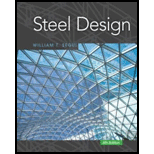# Note For Problems 9.6-1 through 9.6-5, use the lower-bound moment of inertia for deflection of the composite section. Compute this as illustrated in Example 9.7. 9.6-1 Compute the following deflections for the beam in Problem 9.2-1. a. Maximum deflection before the concrete has cured. b. Maximum total deflection after composite behavior has been attained.### Steel Design (Activate Learning wi...

6th Edition
Segui + 1 other
Publisher: Cengage Learning
ISBN: 9781337094740

#### Solutions

Chapter
Section### Steel Design (Activate Learning wi...

6th Edition
Segui + 1 other
Publisher: Cengage Learning
ISBN: 9781337094740
Chapter 9, Problem 9.6.1P
Textbook Problem
5 views

## Note For Problems 9.6-1 through 9.6-5, use the lower-bound moment of inertia for deflection of the composite section. Compute this as illustrated in Example 9.7.9.6-1 Compute the following deflections for the beam in Problem 9.2-1.a. Maximum deflection before the concrete has cured.b. Maximum total deflection after composite behavior has been attained.

To determine

(a)

Maximum deflection before the concrete has cured.

### Explanation of Solution

Given:

A 14X22 composite beam, thickness, t = 4 inches, effective width, b = 90 inches, spacing = 7.5 ft, span length, L = 30 feet.

Construction load = 20 psf, partition load = 10 psf, weight of ceiling and light fixtures = 5 psf, live load = 60 psf.

The value of fc'=4ksi.

Calculation:

The loading condition on the beam before the concrete has cured is as follows:

The self- weight of slab

WS=γC×t12

Where, the thickness of the slab is t=4.0in and the unit weight of concrete is γC=150pcf

WS=150pcf×4.0in12

WS=50.00pcf

WD=(WS×Lp)+W

Where, W is the self- weight of the beam and Lp is the distance between the two adjacent beams.

W=22lbft, as per the manual of AISC.

Substitute the values in the above equation, we get:

WD=(50.00psf×7.5ft)+22lbftWD=(375)+22lbftWD=397lbft.

The live load on the beam before the concrete has cured as follows:

WL=(WSL×LP)

Where, the construction load on the slab is WSL and the span is LP.

WL=(20×7.5)lbftWL=(150)lbft

From the AISC manual for the moment of inertia :

We have, IX=199in4

 DesignationImperial (in x lb/ft) Depthh (in) Widthw (in) Web Thicknesstw (in) Flange Thicknesstf (in) Sectional Area (in2) Weight (lbf/ft) Static Parameters Moment of Inertia Elastic Section Modulus Ix (in4) Iy (in4) Sx (in3) Sy (in3) W 14 x 22 13
To determine

(b)

Maximum total deflection after composite behavior has been attained.

### Still sussing out bartleby?

Check out a sample textbook solution.

See a sample solution

#### The Solution to Your Study Problems

Bartleby provides explanations to thousands of textbook problems written by our experts, many with advanced degrees!

Get Started

Find more solutions based on key concepts
What is atmospheric pressure?

Engineering Fundamentals: An Introduction to Engineering (MindTap Course List)

What is a DBMS? Briefly describe the components of a DBMS.

Systems Analysis and Design (Shelly Cashman Series) (MindTap Course List)

Describe four uses of the combination set.

Precision Machining Technology (MindTap Course List)

digital certificate a. compromised computer or device whose owner is unaware the computer or device is being co...

Enhanced Discovering Computers 2017 (Shelly Cashman Series) (MindTap Course List)

Describe an ideal tack weld.

Welding: Principles and Applications (MindTap Course List)

If your motherboard supports ECC DDR3 memory, can you substitute non-ECC DDR3 memory?

A+ Guide to Hardware (Standalone Book) (MindTap Course List)